# 1195 You Can Find the Answer in This Book

The new school year is underway. Much may have been forgotten over the summer. If you don’t quite remember all the multiplication tables, this puzzle book can help you remember them AND help your brain grow. You might still find it a challenge, but that only makes it more fun!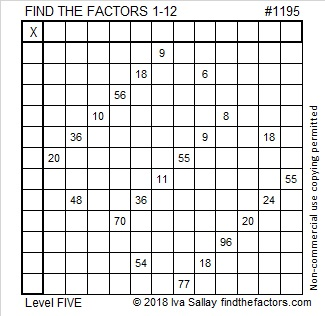Print the puzzles or type the solution in this excel file: 12 factors 1187-1198

Now I’ll share a few facts about the number 1195:

• 1195 is a composite number.
• Prime factorization: 1195 = 5 × 239
• The exponents in the prime factorization are 1 and 1. Adding one to each and multiplying we get (1 + 1)(1 + 1) = 2 × 2 = 4. Therefore 1195 has exactly 4 factors.
• Factors of 1195: 1, 5, 239, 1195
• Factor pairs: 1195 = 1 × 1195 or 5 × 239
• 1195 has no square factors that allow its square root to be simplified. √1195 ≈ 34.568771195 is also the hypotenuse of a Pythagorean triple:
717-956-1195 which is (3-4-5) times 239

# 1167 and Level 5

Will some of the tricky clues in this level 5 puzzle fool you? They won’t if you only write factors of which you are 100% sure. Always use logic. Never guess and check.Print the puzzles or type the solution in this excel file: 12 factors 1161-1173

Now I’ll write a little bit about the number 1167:

• 1167 is a composite number.
• Prime factorization: 1167 = 3 × 389
• The exponents in the prime factorization are 1 and 1. Adding one to each and multiplying we get (1 + 1)(1 + 1) = 2 × 2 = 4. Therefore 1167 has exactly 4 factors.
• Factors of 1167: 1, 3, 389, 1167
• Factor pairs: 1167 = 1 × 1167 or 3 × 389
• 1167 has no square factors that allow its square root to be simplified. √1167 ≈ 34.16138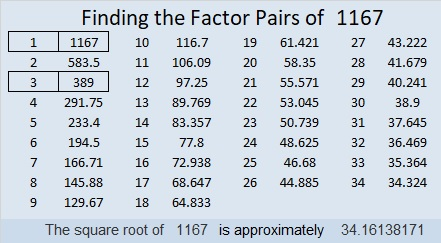1167 is the hypotenuse of a Pythagorean triple:
567-1020-1167 which is 3 times (189-340-389)

1167 is palindrome 5D5 in BASE 14 (D is 13 base 10)
because 5(14²) + 13(14) + 5(1) = 1167

# 1154 and Level 5

I’m sure you can have a lot of fun solving this puzzle. Remember to use logic before you write down any of the factors, and it will be fun instead of frustrating.Print the puzzles or type the solution in this excel file: 10-factors-1148-1160

Now I’ll share some facts about the number 1154:

• 1154 is a composite number.
• Prime factorization: 1154 = 2 × 577
• The exponents in the prime factorization are 1 and 1. Adding one to each and multiplying we get (1 + 1)(1 + 1) = 2 × 2 = 4. Therefore 1154 has exactly 4 factors.
• Factors of 1154: 1, 2, 577, 1154
• Factor pairs: 1154 = 1 × 1154 or 2 × 577
• 1154 has no square factors that allow its square root to be simplified. √1154 ≈ 33.970581154 is the sum of eight consecutive prime numbers:
127 + 131 + 137 + 139 + 149 + 151 + 157 + 163 = 1154

25² + 23² = 1154

1154 is the hypotenuse of a Pythagorean triple:
96-1150-1154 calculated from 25² – 23², 2(25)(23), 25² + 23²

1154 is palindrome 202 in BASE 24
because 2(24²) + 2(1) = 2(24² + 1) = 2(577) = 1154

Did you notice that 1154 has a relationship with 23², 24², and 25²?

# 1142 and Level 5

If you start in the right place, you will be able to solve this puzzle using logic and basic multiplication facts. Don’t get discouraged, if you make a mistake. I know you can figure this one out!Print the puzzles or type the solution in this excel file: 12 factors 1134-1147

Here are some facts about the number 1142:

• 1142 is a composite number.
• Prime factorization: 1142 = 2 × 571
• The exponents in the prime factorization are 1 and 1. Adding one to each and multiplying we get (1 + 1)(1 + 1) = 2 × 2 = 4. Therefore 1142 has exactly 4 factors.
• Factors of 1142: 1, 2, 571, 1142
• Factor pairs: 1142 = 1 × 1142 or 2 × 571
• 1142 has no square factors that allow its square root to be simplified. √1142 ≈ 33.79349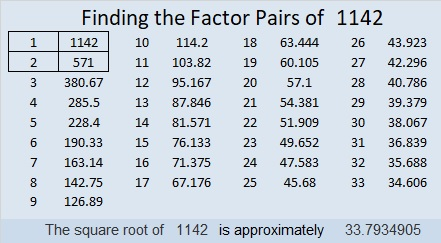1142 is a palindrome in three different bases:
It’s 949 in BASE 11 because 9(11²) + 4(11) + 9(1) = 1142,
3G3 in BASE 17 (G is 16 base 10) because 3(17²) + 16(17) + 3(1) = 1142,
and 2H2 in BASE 20 (H is 17 base 10) because 2(20²) + 17(20) + 2(1) = 1142

# 1127 and Level 5

If the clues in this puzzle were in a Find the Factors 1 – 12, puzzle, the needed factors might be completely different than the ones in this puzzle’s solution. Fortunately, we can only use factors from 1 to 10, so this puzzle will make you think, but shouldn’t be so difficult.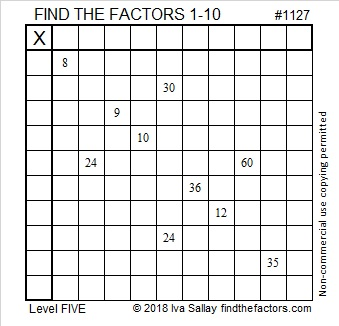Print the puzzles or type the solution in this excel file: 10-factors-1121-1133

Here are a few facts about the number 1127:

• 1127 is a composite number.
• Prime factorization: 1127 = 7 × 7 × 23, which can be written 1127 = 7² × 23
• The exponents in the prime factorization are 2 and 1. Adding one to each and multiplying we get (2 + 1)(1 + 1) = 3 × 2  = 6. Therefore 1127 has exactly 6 factors.
• Factors of 1127: 1, 7, 23, 49, 161, 1127
• Factor pairs: 1127 = 1 × 1127, 7 × 161, or 23 × 49
• Taking the factor pair with the largest square number factor, we get √1127 = (√49)(√23) = 7√23 ≈ 33.570821127 is palindrome 5115 in BASE 6 because 5(6³) + 1(6²) + 1(6) + 5(1) = 1127

# 1117 and Level 5

Can you solve this Level 5 puzzle or will it make you feel like toast? Seriously, I think it’s a little easier than the previous puzzle, so give it a try. You might just become the toast of the town!Print the puzzles or type the solution in this excel file: 12 factors 1111-1119

Now I’ll share some information about the number 1117:

• 1117 is a prime number.
• Prime factorization: 1117 is prime.
• The exponent of prime number 1117 is 1. Adding 1 to that exponent we get (1 + 1) = 2. Therefore 1117 has exactly 2 factors.
• Factors of 1117: 1, 1117
• Factor pairs: 1117 = 1 × 1117
• 1117 has no square factors that allow its square root to be simplified. √1117 ≈ 33.42155

How do we know that 1117 is a prime number? If 1117 were not a prime number, then it would be divisible by at least one prime number less than or equal to √1117 ≈ 33.4. Since 1117 cannot be divided evenly by 2, 3, 5, 7, 11, 13, 17, 19, 23, 29 or 31, we know that 1117 is a prime number.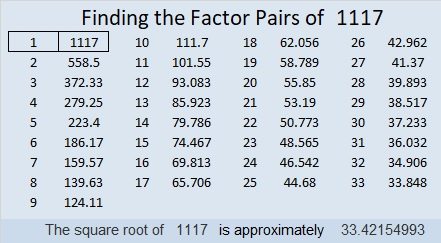26² + 21² = 1117

1117 is the hypotenuse of a Pythagorean triple:
235-1092-1117 calculated from 26² – 21², 2(26)(21), 26² + 21²

Here’s another way we know that 1117 is a prime number: Since its last two digits divided by 4 leave a remainder of 1, and 26² + 21² = 1117 with 26 and 21 having no common prime factors, 1117 will be prime unless it is divisible by a prime number Pythagorean triple hypotenuse less than or equal to √1117 ≈ 33.4. Since 1117 is not divisible by 5, 13, 17, or 29, we know that 1117 is a prime number.

1117 is palindrome 151 in BASE 31 because 1(31²) + 5(31) + 1(1) = 1117

# 1107 and Level 5

Some of this puzzle might be a little tricky, but you won’t allow it to trick you, right? Of course not!Print the puzzles or type the solution in this excel file: 10-factors-1102-1110

Let me tell you something about the number 1107:

• 1107 is a composite number.
• Prime factorization: 1107 = 3 × 3 × 3 × 41, which can be written 1107 = 3³ × 41
• The exponents in the prime factorization are 1 and 3. Adding one to each and multiplying we get (3 + 1)(1 + 1) = 4 × 2 = 8. Therefore 1107 has exactly 8 factors.
• Factors of 1107: 1, 3, 9, 27, 41, 123, 369, 1107
• Factor pairs: 1107 = 1 × 1107, 3 × 369, 9 × 123, or 27 × 41
• Taking the factor pair with the largest square number factor, we get √1107 = (√9)(√123) = 3√123 ≈ 33.271611107 is the hypotenuse of a Pythagorean triple:
243-1080-1107 which is 27 times (9-40-41)

# 1099 and Level 5

The allowable common factors fro 8 and 24 are 2, 4, and 8. Which one of those should you choose? Find a different place to start the puzzle and you shouldn’t have to guess and check to see if you were right.Print the puzzles or type the solution in this excel file: 12 factors 1095-1101

Here are some facts about the number 1099:

• 1099 is a composite number.
• Prime factorization: 1099 = 7 × 157
• The exponents in the prime factorization are 1 and 1. Adding one to each and multiplying we get (1 + 1)(1 + 1) = 2 × 2 = 4. Therefore 1099 has exactly 4 factors.
• Factors of 1099: 1, 7, 157, 1099
• Factor pairs: 1099 = 1 × 1099 or 7 × 157
• 1099 has no square factors that allow its square root to be simplified. √1099 ≈ 33.151171099 = 1 + 0 + 999 + 99. Thank you Stetson.edu for that fun fact.

1099 is the sum of the 13 prime numbers from 59 to 109.
That’s a fact that would take little effort to memorize!

1099 is also the sum of these prime numbers:
139 + 149 +  151 + 157 + 163 + 167 + 173 = 1099
359 + 367 + 373 = 1099

1099 is the hypotenuse of a Pythagorean triple:
595-924-1099 which is 7 times (85-132-157)

1099 is repdigit 777 in BASE 12 because 7(12² + 12 + 1) = 7(157) = 1099
1099 is palindrome 4D4 in BASE 15 (D is 13 in base 10)
because 4(15²) + 13(15) + 4(1) = 1099

# 1091 and Level 5

Can you figure out where to put all the numbers from 1 to 10 in both the first column and the top row so that those factors and the clues can become a multiplication table? Some of the clues might be a little tricky, but I’m sure you can figure them all out.Print the puzzles or type the solution in this excel file: 10-factors-1087-1094

Here are a few facts about the number 1091:

• 1091 is a prime number.
• Prime factorization: 1091 is prime.
• The exponent of prime number 1091 is 1. Adding 1 to that exponent we get (1 + 1) = 2. Therefore 1091 has exactly 2 factors.
• Factors of 1091: 1, 1091
• Factor pairs: 1091 = 1 × 1091
• 1091 has no square factors that allow its square root to be simplified. √1091 ≈ 33.03029

How do we know that 1091 is a prime number? If 1091 were not a prime number, then it would be divisible by at least one prime number less than or equal to √1091 ≈ 33. Since 1091 cannot be divided evenly by 2, 3, 5, 7, 11, 13, 17, 19, 23, 29 or 31, we know that 1091 is a prime number.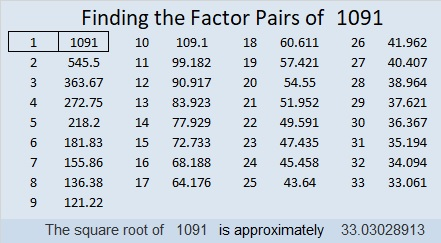1091 is the first prime number in the prime triplet (1091, 1093, 1097). It is also the middle number in the prime triplet (1087, 1091, 1093).

1091 looks interesting when it is written in some other bases:
It’s 13331 in BASE 5 because 1(5⁴) + 3(5³) + 3(5²) + 3(5) + 1(1) = 1091,
3D3 in BASE 17 (D is 13 base 10) because 3(17²) + 13(17) + 3(1) = 1091,
and it’s 123 in BASE 32 because 1(32²) + 2(32) + 3(1) = 1091

# 1085 and Level 5

Here’s another tricky level 5 puzzle for you to solve. Use logic, not guess and check, and you’ll do great!Print the puzzles or type the solution in this excel file: 12 factors 1080-1086

Here are a few facts about the number 1085:

• 1085 is a composite number.
• Prime factorization: 1085 = 5 × 7 × 31
• The exponents in the prime factorization are 1, 1, and 1. Adding one to each and multiplying we get (1 + 1)(1 + 1)(1 + 1) = 2 × 2 × 2 = 8. Therefore 1085 has exactly 8 factors.
• Factors of 1085: 1, 5, 7, 31, 35, 155, 217, 1085
• Factor pairs: 1085 = 1 × 1085, 5 × 217, 7 × 155, or 31 × 35
• 1085 has no square factors that allow its square root to be simplified. √1085 ≈ 32.93934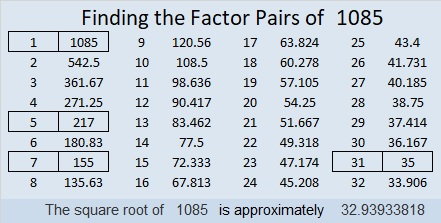31 × 35 = 1085 means we are  only 4 numbers away from the next perfect square and
33² – 2² = 1085

1085 is the hypotenuse of a Pythagorean triple:
651-868-1085 which is (3-4-5) times 217

1085 looks interesting when it is written using a different base:
It’s 5005 in BASE 6 because 5(6³ + 1) = 1085,
765 in BASE 12 because 7(144) + 6(12) + 5(1) = 1085,
656 in BASE 13 because 6(13²) + 5(13) + 6(1) = 1085
VV in BASE 34 (V is 31 base 10) because 31(34) + 31(1) = 1085, and
it’s V0 in BASE 35 because 31(35) = 1085